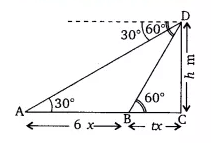Q

# A straight highway leads to the foot of a tower. A man standing at the top of the tower observes a car at an angle of depression of 30°, which is approaching the foot of the tower with a uniform speed. Six seconds later, the angle of depression of the car

15. A straight highway leads to the foot of a tower. A man standing at the top of the tower observes a car at an angle of depression of 30°, which is approaching the foot of the tower with a uniform speed. Six seconds later, the angle of depression of the car is found to be 60°. Find the time taken by the car to reach the foot of the tower from this point.

ViewsLet  be the height of the tower (DC) and the speed of the car be . Therefore, the distance (AB)covered by the car in 6 seconds is 6 m. Let  time required to reach the foot of the tower. So, BC =

According to question,
In triangle ,
..........................(i)

In triangle ,

...................(ii)

Put the value of  in equation (i) we get,

Hence, from point B car take 3 sec to reach the foot of the tower.

Exams
Articles
Questions